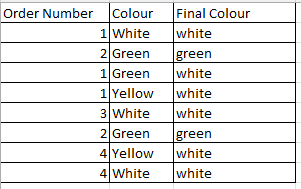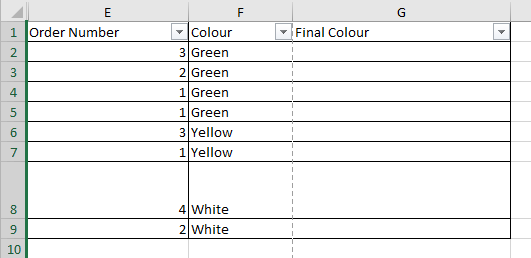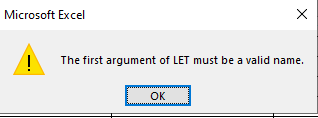# Excel Automation> Comparing 2 column and write final value in another column

I want to put value in column C/Final color wrt column A/order number and column B/colour.
For e.g.

1. same order number will have same color in final color column.
2. But final color value in Final color column for respective ON depends on preference of color Preference: (White>Yellow>Green)
which means if one value for ON 1 is yellow and one value for ON1 is white, we will give preference to white and write same in Final color column value.

PFA snip for referenceWould prefer if someone can provide a excel formula for same instead of gng via UiPath. Thanks in advance.

Hello @anjasing

Assuming you have a DataTable named “dataTable” with columns “ON” and “Preference,” and you want to calculate “FinalColor” in column C

For Each row As DataRow In dataTable.Rows
Dim orderNumber As String = row(“ON”).ToString()
Dim preference As String = row(“Preference”).ToString()
Dim finalColor As String = “”

``````If preference = "White" Then
finalColor = "White"
ElseIf preference = "Yellow" Then
finalColor = "Yellow"
ElseIf preference = "Green" Then
finalColor = "Green"
End If

Assign the calculated final color to the "FinalColor" column in the same row
row("FinalColor") = finalColor
``````

Next

Thanks & Cheers!!!

hey! column Names are being showed in image itself. There is no other column except that.
Also, is there any way of excel formula instead of going via UiPath?

Hello @anjasing,

with Excelforumulas you could do it like this:

=LET(Matrixvals;CHOOSECOLS(UNIQUE(FILTER(\$A\$2:\$B\$9;\$A\$2:\$A\$9=A2);FALSE;FALSE);2);
IFS(
ISNUMBER(MATCH(“white”;Matrixvals;0));“white”;
ISNUMBER(MATCH(“yellow”;Matrixvals;0));“yellow”;
ISNUMBER(MATCH(“green”;Matrixvals;0));“green”;
TRUE;“n/a”
))

Kind Regards

1 Like

HI @lukas.gamper
Thanks for providing a solution.
Actually having one issue.
this is the real excel i am working onand I am giving below formula:

=LET(Matrixvals;CHOOSECOLS(UNIQUE(FILTER(\$E\$2:\$F\$9;\$E\$2:\$E\$9=E2);FALSE;FALSE);6);
IFS(
ISNUMBER(MATCH(“White”;Matrixvals;0));“White”;
ISNUMBER(MATCH(“Yellow”;Matrixvals;0));“Yellow”;
ISNUMBER(MATCH(“Green”;Matrixvals;0));“Green”;
TRUE;“n/a”
))

Please correct me if I am wrong. As it’s giving issue.=LET(Matrixvals;CHOOSECOLS(UNIQUE(FILTER(\$E\$2:\$F\$9;\$E\$2:\$E\$9=E2);FALSE;FALSE);2) ← should still be a 2 not 6. It takes the 2nd column of the defined matrix, not the 6 column in your excel

1 Like

=LET(Matrixvals;CHOOSECOLS(UNIQUE(FILTER(\$E\$2:\$F\$9;\$E\$2:\$E\$9=E2);FALSE;FALSE);2);
IFS(
ISNUMBER(MATCH(“White”;Matrixvals;0));“White”;
ISNUMBER(MATCH(“Yellow”;Matrixvals;0));“Yellow”;
ISNUMBER(MATCH("Green”;Matrixvals;0));“Green”;
TRUE;“n/a”
))

this is correct right??
BUt it’s still giving same issue

should be correct…

=LET(Matrixvals;CHOOSECOLS(UNIQUE(FILTER(\$E\$2:\$F\$9;\$E\$2:\$E\$9=E2);FALSE;FALSE);2);

IFS(

ISNUMBER(MATCH(“white”;Matrixvals;0));“white”;

ISNUMBER(MATCH(“yellow”;Matrixvals;0));“yellow”;

ISNUMBER(MATCH(“green”;Matrixvals;0));“green”;

TRUE;“n/a”

))

which version of Excel do you use?

Kind Regards

1 Like

I am not a 100% certain, but could it be you need to replace ; → with , because of language settings?for all the semicolon it should be replaced?

if you type excel formulas, do you normally use , or ;. Because I have seen both, if you need to use , you need to replace all. If you use ; anyway, then it’s not the issue of ; or ,.

Since I work with DE Excel and Formulas, not the EN version1 Like

MatrixExcel.xlsx (11.5 KB)

maybe you can just use the excel here and it will convert correctly?

oh got it,
we use , only
I guess that’s language issue only.

1 Like

=LET(Matrixvals,CHOOSECOLS(UNIQUE(FILTER(\$A\$2:\$B\$9,\$A\$2:\$A\$9=A2),FALSE,FALSE),2),
IFS(
ISNUMBER(MATCH(“White”,Matrixvals,0)),“White”,
ISNUMBER(MATCH(“Yellow”,Matrixvals,0)),“Yellow”,
ISNUMBER(MATCH(“Green”,Matrixvals,0)),“Green”,
TRUE,“n/a”
))

=LET(Matrixvals,CHOOSECOLS(UNIQUE(FILTER(\$A\$2:\$B\$9,\$A\$2:\$A\$9=A2),FALSE,FALSE),2),
IFS(
ISNUMBER(MATCH(“White”,Matrixvals,0)),“White”,
ISNUMBER(MATCH(“Yellow”,Matrixvals,0)),“Yellow”,
ISNUMBER(MATCH(“Green”,Matrixvals,0)),“Green”,
TRUE,“n/a”
))

does work?

this issue

the only difference now is " and ” I guess, DE and EN version also need the right "

had to change , to ;, so the formula would even work, but if i change " to ” I get # NAME? error. So I think it’s that

You were absolutely correct. Thank you so much for constantly helping out. That means a lot. Thank youIt’s working now.

1 Like

This topic was automatically closed 3 days after the last reply. New replies are no longer allowed.